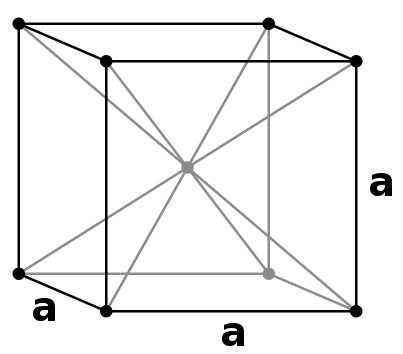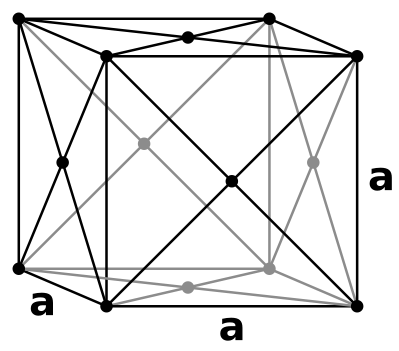# 1.1: The Unit Cell

$$\newcommand{\vecs}{\overset { \rightharpoonup} {\mathbf{#1}} }$$ $$\newcommand{\vecd}{\overset{-\!-\!\rightharpoonup}{\vphantom{a}\smash {#1}}}$$$$\newcommand{\id}{\mathrm{id}}$$ $$\newcommand{\Span}{\mathrm{span}}$$ $$\newcommand{\kernel}{\mathrm{null}\,}$$ $$\newcommand{\range}{\mathrm{range}\,}$$ $$\newcommand{\RealPart}{\mathrm{Re}}$$ $$\newcommand{\ImaginaryPart}{\mathrm{Im}}$$ $$\newcommand{\Argument}{\mathrm{Arg}}$$ $$\newcommand{\norm}{\| #1 \|}$$ $$\newcommand{\inner}{\langle #1, #2 \rangle}$$ $$\newcommand{\Span}{\mathrm{span}}$$ $$\newcommand{\id}{\mathrm{id}}$$ $$\newcommand{\Span}{\mathrm{span}}$$ $$\newcommand{\kernel}{\mathrm{null}\,}$$ $$\newcommand{\range}{\mathrm{range}\,}$$ $$\newcommand{\RealPart}{\mathrm{Re}}$$ $$\newcommand{\ImaginaryPart}{\mathrm{Im}}$$ $$\newcommand{\Argument}{\mathrm{Arg}}$$ $$\newcommand{\norm}{\| #1 \|}$$ $$\newcommand{\inner}{\langle #1, #2 \rangle}$$ $$\newcommand{\Span}{\mathrm{span}}$$$$\newcommand{\AA}{\unicode[.8,0]{x212B}}$$

The Unit Cell refers to a part of a simple crystal lattice, a repetitive unit of solid, brick-like structures with opposite faces, and equivalent edge points. In 1850, Auguste Bravais proved that crystals could be split into fourteen unit cells. Although there are several types of unit cells found in cubic lattices, we will be discussing the basic ones: Simple Cubic, Body-centered Cubic, and Face-centered Cubic. If any atom recrystalizes, it will eventually become the original lattice. Crystallization refers the purification processes of molecular or structures;.

## Introduction

The Unit Cell contains seven crystal systems and fourteen crystal lattices. These unit cells are given types and titles of symmetries, but we will be focusing on cubic unit cells. One of the most commonly known unit cells is rock salt NaCl (Sodium Chloride), an octahedral geometric unit cell. The whole lattice can be reproduced when the unit cell is duplicated in a three dimensional structure. These unit cells are imperative for quite a few metals and ionic solids crystallize into these cubic structures. Calculating with unit cells is a simple task because edge-lengths of the cell are equal along with all 90⁰ angles.

## Simple Cubic Unit CellsSimple Cubic unit cells indicate when lattice points are only at the corners. They are the simplest (hence the title) repetitive unit cell. The lattice points at the corners make it easier for metals, ions, or molecules to be found within the crystalline structure. This phenomena is rare due to the low packing of density, but the closed packed directions give the cube shape.

Since the edges of each unit cell are equidistant, each unit cell is identical. In order to be labeled as a "Simple Cubic" unit cell, each eight cornered same particle must at each of the eight corners. This unit cell only contains one atom. Its packing efficiency is about 52%.

To packing efficiency, we multiply eight corners by one-eighth (for only one-eighth of the atom is part of each unit cell), giving us one atom.

8 Corners of a given atom x 1/8 of the given atom's unit cell = 1 atom

To calculate edge length in terms of r the equation is as follows:

2r

An example of a Simple Cubic unit cell is Polonium.

## Body-centered Cubic Unit Cells

Body-centered Cubic (BCC) unit cells indicate where the lattice points appear not only at the corners but in the center of the unit cell as well. The atoms touch one another along the cube's diagonal crossing, but the atoms don't touch the edge of the cube. All atoms are identical. This type of unit cell is more common than that of the Simple Cubic unit cell due to tightly packed atoms. Its packing efficiency is about 68% compared to the Simple Cubic unit cell's 52%.This unit cells contains two atoms.

To determine this, we multiply the previous eight corners by one-eighth and add one for the additional lattice point in the center.

(8 Corners of a given atom x 1/8 of the given atom's unit cell) + 1 additional lattice point = 2 atoms)

To calculate edge length in terms of r the equation is as follows:

$\dfrac{4r}{\sqrt{3}}$

Some examples of BCCs are Iron, Chromium, and Potassium.

It is a common mistake for CsCl to be considered bcc, but it is not. Instead, it is non-closed packed.

## Face-centered Cubic Unit Cells

Face-centered Cubic (FCC) unit cells indicate where the lattice points are at both corners and on each face of the cell.This is a more common type of unit cell since the atoms are more tightly packed than that of a Simple Cubic unit cell. Like the BCC, the atoms don't touch the edge of the cube, but rather the atoms touch diagonal to each face. Its packing efficiency is the highest with a percentage of 74%. Atoms touch one another along the face diagonals. All atoms are identical.

This unit cell contains four atoms.

To determine this, we take the equation from the aforementioned Simple Cubic unit cell and add to the parenthesized six faces of the unit cell multiplied by one-half (due to the lattice points on each face of the cubic cell).

(8 corners of a given atom x 1/8 of the given atom's unit cell) + (6 faces x 1/2 contribution) = 4 atoms)

To calculate edge length in terms of r the equation is as follows:

$2 \sqrt{2}r$

## References

1. Brown, Theodore L. Chemistry: the Central Science. 10th ed. Upper Saddle River, NJ: Pearson Prentice Hall, 2006. Print. (Pgs 466-467)
2. Housecroft, Catherine E., and Alan G. Sharpe. Inorganic Chemistry. 3rd ed. Harlow: Pearson Education, 2008. Print. (Pgs 149-151)
3. Tro, Nivaldo J. Chemistry: a Molecular Approach. 1st ed. Upper Saddle River, NJ: Pearson/Prentice Hall, 2008. Print. (Pgs 498-500)